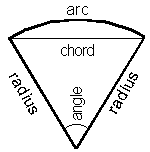Cleave Books
The Sectors Calculator
 Show values to . . . 3 4 5 6 7 8 9 significant figures.
 radius units angle degreesarc units chord units area square units
Remember: Appropriate units need to be attached.
Very large and very small numbers appear in e-Format.
Unvalued zeros on all numbers have been suppressed.
The original inputs have NOT been adjusted in any way.
A note on Format and Accuracy is available.

 Additional Information A sector (of a circle) is made by drawing two lines from the centre of the circle to the circumference, and it looks like the usual 'wedge' cut from a cake.There are two special cases.If the angle is 180 degrees then the sector is a semi-circle.If the angle is 360 degrees then the sector is a full circle.Clearly the angle cannot be greater than 360 degrees.Where (for brevity) it says 'radius', 'arc' and so on, it should, more correctly, be something like 'length of radius' or 'arc-length' etc, and 'angle' means 'angle at the centre'. A problem not dealt with by this calculator is where the length of the chord (c) and the height (h) between the chord and arc are known, and it is required to find the radius (r).r   =  (c² / 8h) + (h / 2)In words: c squared divided by 8h plus (h divided by 2)If the perimeter is needed, add together the lengths ofradius + radius + arc Caution. Do NOT confuse a 'sector' with a 'segment'.A segment is the shape formed between the chord and the arc.
 LimitationsIn the more extreme cases the accuracy falls off.This can arise when using very small or very large values, or when the difference between the two given values (say the chord and the arc) is very small.In those cases, the solutions offered must be treated with some caution if considerable accuracy is necessary. This is due to the intrepretation of the sine function within the browser.The message "This case cannot be resolved" only means that it cannot be done within the limits of this calculator. It does NOT mean that a solution is impossible.Given the area & chord, two solutions are often possible. Only one is given here.

Go to Cleave Books main Index